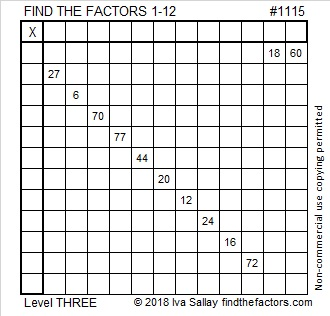# 1115 and Level 3

You will have to know the 11 and 12 times tables to solve this Level 3 Find the Factors 1-12 puzzle, but I’m sure you can do it! Stick with it, and don’t give up! Start with the clues at the top of the puzzle and work down row by row until it’s completed.Print the puzzles or type the solution in this excel file: 12 factors 1111-1119

1115 is not a clue just the puzzle number. Here are some facts about the number 1115:

• 1115 is a composite number.
• Prime factorization: 1115 = 5 × 223
• The exponents in the prime factorization are 1 and 1. Adding one to each and multiplying we get (1 + 1)(1 + 1) = 2 × 2 = 4. Therefore 1115 has exactly 4 factors.
• Factors of 1115: 1, 5, 223, 1115
• Factor pairs: 1115 = 1 × 1115 or 5 × 223
• 1115 has no square factors that allow its square root to be simplified. √1115 ≈ 33.391621115 is the sum of nine consecutive prime numbers:
103 + 107 + 109 + 113 + 127 + 131 + 137 + 139 + 149 = 1115

1115 is the hypotenuse of a Pythagorean triple:
669-892-1115 which is (3-4-5) times 223

1115 is palindrome 2B2 in BASE 21 (B is 11 base 10)
because 2(21²) + 11(21) + 2(1) = 1115

This site uses Akismet to reduce spam. Learn how your comment data is processed.